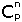Home > Palm > Samples > CombinationCombination

#### What's that?

Remember those lessons of statistics you had long ago? Factorial? Combination? Well, we'll take those formulas to show how you can develop a mobile application using various SDK's and tools.

#### Factorial

Let's say we have letters A, B and C. How many "words" can we make, using each letter only once. By word, we mean "any series of three letters, even if it's not in a dictionary". ACB is one of them. Let's list all of'em: ABC, ACB, BAC, BCA, CAB, CBA. Another way to look at it is to say:

1. For the first letter, I can pick A, B or C, which means I have 3 possibilities. Let me pick A.
2. Then, for the second letter, I can only pick B or C, which means 2 possibilities. I pick B
3. Finally, the only option left for the last letter is C: 1 possilibity

The total number of words is 6: three possibilities multiplied by two possibilities then by one. Hey that works! Look at our enumeration of words a bit above! Great... We just illustrated how to calculate a factorial: factorial(3)=3x2x1=6. You'll write this as 3! = 6.

4! = 4x3x2x1 = 4 x (3x2x1)= 4 x 3!
5! = 5x4x3x2x1 = 5 x (4x3x2x1) = 5 x 4!
1000! = 1000x999x998x997x...x1 = 1000 x 999!, or as a general definition:
n! = n x (n-1)!

#### Combination

Now, let's take another example. You would like to win the prize of this \$1000 lottery. Here's the rule: there are five balls numbered 1 thru 5 and you must pick two of them. How many combinations are there? Well, go back to our previous example and apply the same principle here:

1. For the first ball, I have 5 choices
2. For the second one, I only have 4 choices
3. For the third one... Hey, wait a minute, there is NO such "third one". You are allowed to pick only two numbers!

The total number of choices is 5 x 4 = 20.

Really? Is is THAT simple? Well, not quite! Let's see why. Here's a list of all the combinations:

• 1 2
• 1 3
• 1 4
• 1 5
• 2 1... Er... Really?!? No way!!! Actually, in any lottery, "1 and 2" is same as "2 and 1"
• 2 3... This one is okay, let's continue on without repeating choices as in previous bullet
• 2 4
• 2 5
• 3 4
• 3 5
• 4 5

In other words, ORDER doesn't count. Well, it's a good thing for us whenever we buy a lottery ticket, as this gives us the chance to win if the winner is "ball 5 and ball 2" and we chose "ball 5 and ball 2".

So, we'll have to divide our "5 x 4 = 20" from above by 2. If we had to pick 3 balls, we would divide 5 x 4 x 3 by 3 x 2, because there are 3x2 ways of making a "three-letter word out of three given letters".

You will notice that 5 x 4 = (5 x 4 x 3 x 2 x 1) / (3 x 2 x 1) = 5! / 3!

The final result is 5! / 3! / 2! = 10. Check this formula against the list above.

You probably know that 3 = 5 - 2.

Remember? 5 is the total number of balls and 2 is how many of'em we can pick. The general formula to calculate the number of combinations of n items taken by p at a time is:= n! / (n-p)! / p! = n! / [(n-p)! x p!]

#### Our sample

This small app implements three modules:

1. factorial
2. combination, which calls factorial three times, as the formula states
3. go, which calls factorial(n), factorial(p), factorial(n-p) just to display intermediate values, and finally combination(n, p)

I intentionally didn't optimize this application. In a commercial app, it would make sense to not code the combination formula as is. It'd be smarter to calculate a "partial factorial" than to calculate two factorials and divide them.

Same remark for recursion. We did use recursion whenever the language's implementation allowed it. Again, a simple loop would run quicker and save stack space, which is very valuable on our small devices :)